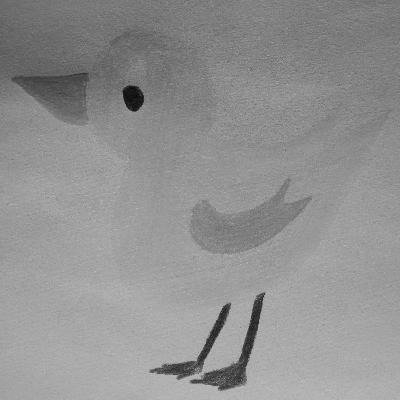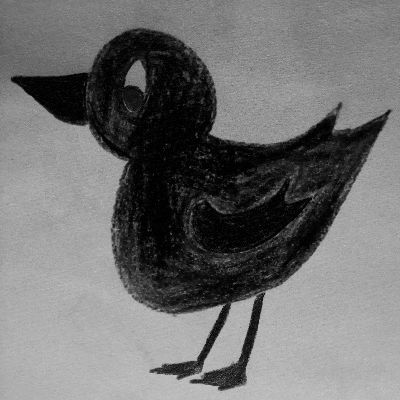# Adaptive RGB to Grayscale Direct Conversion for Binary Thresholding

I’ve been working on a project in which conversion of colored images to grayscale forms was needed. Images were drawn stuff on bright backgrounds, so maximum contrast between background and foreground was inevitable. I tested some typical methods and at last, came up with my own adaptive version.

Conversion of an RGB image to grayscale is as simple as merging R, G and B channels together via a weighted average. The point is just to choose the right coefficients so that gray = C₁.R + C₂.G + C₃.B.

This Wikipedia entry has provided various coefficient sets considering different color spaces and features like gamma corrections. But in my case, none of them were suitable enough to provide sufficient separation of fore/background as the step prior to binary thresholding.

As the purpose is to separate the foreground from a brighter background, I calculate the average of pixels above a determined threshold (the algorithms main parameter) so as bright pixels would not be taken into account. By reducing the two largest channels’ final coefficient, according to the average using c_subdiv parameter, we get darker results in the foreground. By normalizing the calculated values, we get a valid result. The following pseudo code presents the idea:

`// every operation on coeffs array is itemwiseAdaptiveGSCoeffs (threshold, c_subdiv):    coeffs = [0, 0, 0]    count = 0    for w from 0 to image_width:        for h from 0 to image_height:            pixel = image[w, h] // 3 r, g and b channels            ch_avg = channels_average(pixel)            if max(                abs(pixel.r - ch_avg),                 abs(pixel.g - ch_avg),                 abs(pixel.b - ch_avg),             ) > threshold:            coeffs += pixel            count += 1                coeffs /= count        coeffs[argmax(coeffs)] /= c_subdiv // first largest    coeffs[argmax(coeffs)] /= c_subdiv // second largest        coeffs = int(coeffs)            coeffs /= coeffs.r + coeffs.g + coeffs.b        return coeffs`

Although, this idea has a drawback which is in images with color of different intensities, brighter colors may not get darken enough.

Consider The Duck as the reference image. Results below show the performance of the presented idea:(1) Simple unweighted average — (2) Luma coefficients — (3) Colorimetric coefficientsMy idea: (1) threshold = 5 , s_subdiv = 5 — (2) threshold = 5 , s_subdiv = ∞

I hope it helps someone somehow!

Software Engineer, Game Programmer, AI M.Sc. Student

## More from Arsham Khashabi

Software Engineer, Game Programmer, AI M.Sc. Student